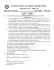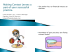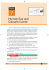# CBSE - Kinematics

## Transcription

CBSE - Kinematics
```http://iitscholars.com http://iitvidya.com http://iitscholar.com
1.
2.
3.
4.
5.
6.
7.
8.
9.
10.
11.
MATHEMATICS
If a line OP through the origin O makes angles , 45°
and 60° with x, y and z axis respectively then the 12.
direction cosines of OP are
(A) 1/ 2, 1/ 2, 1/ 2 (B) 1/2,1/2, 1/ 2
(C) 1/2, 1/ 2 , 1/2
(D) none of these
An equation of z–axis is
(A) z = 0, x = 0
(B) z = 0, y = 0
(C) x = 0, y = 0
(D) x = k, y = –k., (k  0)
The ratio in which the yz plane divides the segment
joining the points (–2, 4, 7) and (3, –5, 8) is
(A) 2 : 3
(B) 3 : 2
(C) 4 : 5
(D) –7 : 8
The coordinates of a point equidistant from the points (a,
0, 0), (0, a, 0), (0, 0, a) and (0, 0, 0) are
(A) (a/3, a/3, a/3)
(B) (a/2, a/2, a/2)
(C) (a, a, a)
(D) (2a, 2a, 2a)
13.
If O is the origin and the line OP of length r makes an
angle  with x–axis and lies in the xz plane, then the
14.
coordinate of P are
(A) (r cos , 0, r sin )
(B) (0, 0, r sin)
(C) (0, 0, r cos)
(D) (r cos, 0, 0)
If a line is equally inclined with the coordinate axes, then
the angle of inclination is
(A) cos–1 (1/2)
(B) cos–1 1 / 2 
(C) cos–1 (1/ 3 )
(D) cos–1( 3 / 2)
A line makes an angle of 60° with each of x and y axis,
the angle which it makes with z axis is
(A) 30°
(B) 45°
15.
(C) 60°
(D) none of these
If a1, a2, … form a G.P. and ai > 0 for all i  1, then
log a m log a m 1 log a m  2
  log a m 3 log a m  4 log a m 5 is equal to
log a m  6 log a m  7 log a m 8
(A) log (am + 8) – log (am)
(B) log (am + 8) + log (am)
(C) zero
16.
(D) log2am+ 4
If the system of equations x + ay + az = 0; bx + y + bz =
0; cx + cy + z = 0, where a, b, c are non–zero and non–
unity has a non–trival solution, then the value of
17.
a
b
c


is
1 a 1 b 1 c
(A) zero
(B) 1
abc
(C) –1
(D) 2
a  b2  c2
x 2  3x x  1 x  3
If px4 + qx3 + rx2 + sx + t = x 2  1 2  x x  3 then 18.
x 2  3 x  4 3x
p is equal to
(A) –5
(B) –4
(C) –3
(D) –2.
If A and B are symmetric matrices then AB – BA is a
(A) symmetric matrix
(B) skew symmetric matrix
(C) diagonal matrix (D) null matrix.
19.
 3
1 


1 1
2 
If P   2
,A
 and Q = P AP' then P'
 1
3
0 1


 2 2 
(Q2005) P is equal to
1 2005
(A) 
1 
0
 3
(B)  2

 0

2005

1 
1 

 
 3

 1
2
1 


(C) 
(D) 2
.


 3

 1 2005
 2 2005
If A is matrix such that A2 + A + 2I = 0, then
(A) A is non-singular (B) A is symmetric
(C) A = 0
(D) none of these
If a, b and c are all different from zero such that
1
1 
1  a
1 1 1

   0 , then the matrix A   1 1  b
1 
a b c
 1
1 1  c 
is
(A) symmetric
(B) singular
(C) a skew symmetric matrix
(D) none of these
Consider the system of equation ax1 + b1y + c1z = 0, a2x
a1 b1 c1
+ b2y + c2z = 0, a3x + b3y + c3z = 0 if a 2 b 2 c2  0,
a 3 b 3 c3
then the system has
(A) infinite number of solutions
(B) only one solution
(C) no solution
(D) none of these
If A and B are two invertible matrices of the same order,
then the adjoint of AB is equal to
(D) none of these
Consider an infinite geometric series with first term a and
common ratio r. If its sum is 4 and the second term is 3/4,
then
7
3
(A) a  , r 
(B) a = 2, r = 3/8
4
7
3
1
(C) a  , r 
(D) a = 3, r = 1/4
2
2
Let S1, S2, … be squares such that for each n  1, the
length of a side of Sn equals the length of the diagonal of
Sn+1. If the length of a side of Si is 10 cm, then for which
of the following values of n is the area of Sn less than 1
sq. cm?
(A) 7
(B) 8
(C) 11
(D) none of these
If cos (x – y), cosx and cos (x + y) are in H.P., then cosx
y
sec   is equal to
2
http://iitscholars.com http://iitvidya.com http://iitscholar.com
http://iitscholars.com http://iitvidya.com http://iitscholar.com
(A) 
(C)  2
20.
21.
22.
23.
24.
25.
26.
27.
28.
29.
30.
2
(B) 
1
31.
2
(D) none of these
If log3 2, log3 (2x – 5) and log3 (2x – 7/2) are in A.P., then
x is equal to
(A) 2
(B) 3
(C) 4
(D) 2, 3
If a, b, c are in arithmetic progression then
1
1
1
are in
,
,
a b
a c
b c
(A) A.P.
(B) G.P.
(C) H.P.
(D) none of these
If (m + 1)th, (n + 1)th and (r + 1)th term of an A.P. are in
G.P. and m, n, r are in H.P., then the ratio of the first term
of the A.P. to its common difference in terms of n is
(A) n/2
(B) –n/2
(C) n/3
(D) –n/3
The number of parallelograms that can be formed from a
set of four parallel lines intersecting another set of three
parallel lines is
(A) 6
(B) 18
(C) 12
(D) 9
A person wishes to make up as many different parties as
he can out of 20 friends, each party consisting of the
same number. The number of persons he should invite at
a time is
(A) 8
(B) 10
(C) 12
(D) none of these.
The number of ways in which 30 marks can be allotted to
8 questions if each question carries at least 2 marks, is
(A) 115280
(B) 117280
(C) 116280
(D) none of these.
In a network of railways, a small island has 15 stations.
The number of different types of tickets to be printed for
each class, if every station must have tickets for other
station, is
(A) 230
(B) 210
(C) 340
(D) none of these.
A father with 8 children takes them 3 at a time to the
zoological garden, as he can without taking the same 3
children together more than once. Then
(A) number of times he will go is 56
(B) number of times each child will go is 21
(C) number of times a particular child will not go is 35
32.
33.
34.
35.
A particle is moving on a straight line, where its position
s, in meters, as a function of time t in seconds is given by
s = t3 + at2 + bt + c where a, b, c are constants. It is
known that at t = 1 sec; the position of the particle is s =
7m, velocity is 7 m/s and acceleration is 12 m/s2. The
values of a, b, c are respectively
(A) 3, 2, 5
(B) -3, 2, 5
(C) 3, -2, 5
(D) none of these
A and B play a game of tennis. The situation of the game
is as follows; if one scores two consecutive points after a
deuce he wins; if loss of a point is followed by win of a
point, it is deuce. The chance of a server to win a point is
2/3. The game is at deuce and A is serving. Probability
that A will win the match is, (serves are changed after
each game)
(A) 3/5
(B) 2/5
(C) 1/2
(D) 4/5
A die is thrown three times and the sum of three numbers
obtained is 15. The probability of first throw being 4 is
(A) 1/18
(B) 1/5
(C) 4/5
(D) 17/18
Six different balls are put in three different boxes, no box
being empty. The probability of putting balls in the boxes
in equal numbers is
(A) 3/10
(B) 1/6
(C) 1/5
(D) none of these
Fifteen coupons are numbered 1, 2, 3, .... 15. Seven
coupons are selected at random one at a time with
replacement. The probability that the largest number
appearing on the selected coupon is 9, is
6
36.
37.
(D) all of these.
38.
A boat is to be manned by eight men of whom 2 can only
row on bow side and 1 can only row on stroke side; the
number of ways in which the crew can be arranged is
(A) 4360
(B) 5760
(C) 5930
(D) none of these.
39.
The number of proper divisors of 2520 is
(A) 46
(B) 52
(C) 64
(D) none of these
A man who swims at the rate of 5 m/s in still water
wishes to cross a river of breadth 20 m flowing at the rate
of 3 m/s. The time taken by man to cross the river is
(A) 8s
(B) 6s
40.
(C) 5s
(D) 4s
7
 9
 8
(A)  
(B)  
 16 
 15 
7
7
9 8
(C)
(D) none of these
157
Three persons A1, A2 and A3 are to speak at a function
along with 5 other persons. If the person speak in random
order, the probability that A1 speaks before A2 and A2
speaks before A3 is
(A) 1/6
(B) 3/5
(C) 3/8
(D) none of these
A fair coin is tossed a fixed number of times. If the
then the probability of getting 2 heads is,
(A) 15/28
(B) 2/15
13
(C) 15/2
(D) none of these
ˆ
ˆ
ˆ
ˆ
If a  i  j  k,b  4i  3jˆ  4kˆ and c  ˆi  ˆj  kˆ are
linearly dependent vectors and | c | = 3 , then
(A)  = 1,  = -1
(B)  = 1,  1
(C)  = -1,   1
(D)  = 1,  = 1
Let a  2iˆ  ˆj  2kˆ and b  ˆi  ˆj . If c is a vector such that

a.c = | c |, | c - a | = 2 2 and the angle between a  b



and c is 30°, then a  b  c is equal to
(A) 2/3
(C) 2
(B) 3/2
(D) 3
Let a  2iˆ  ˆj  kˆ , b  ˆi  2jˆ  kˆ and a unit vector c be
coplanar. If c is perpendicular to a , then c is equal to
http://iitscholars.com http://iitvidya.com http://iitscholar.com
http://iitscholars.com http://iitvidya.com http://iitscholar.com
 ˆj  kˆ 
2
1

41.
42.
43.




1 ˆ ˆ ˆ
i j k
3
1 ˆ ˆ
1 ˆ ˆ ˆ
(C)
(D)
i  2j
i  j k
5
2
51.
The distance in meters described by a particle in t
seconds is given by s = aet + be-t. The acceleration of the
particle at instant t is
52.
(A) s m/s2
(B) ab m/s2
(C) s/(a2 + b2) m/s2
(D) none of these
If a both moves so that the distance sm which it moves in
t seconds is given by the relation s = at3. The time t when
magnitude of the velocity and acceleration are equal, is
53.
(A) 2s
(B) 3s
(C) 4s
(D) none of these
If
and
are
unit
vectors,
then
c
a, b
(A)
2
(B)

2
2
a  b  b  c  c  a does not exceed
(A) 4
(C) 8
44.
45.
46.
47.
(B) 9
(D) 6
If a  r  b  ta and a.r  3, where a  2iˆ  ˆj  kˆ and
b  ˆi  2jˆ  kˆ then r equals
7 2
7
1
(A) ˆi  ˆj
(B) ˆi  ˆj
6 5
6
3
7ˆ 2ˆ 1 ˆ
(C) i  j  k
(D) none of these
6 3 3
If | a  b |  | a |, then b.(2a  b) equals
(A) 0
(B) 1
(C) 2a.b
(D) none of these
Two equal forces P and P act at a point and their resultant
is P. The angle between the forces is
(A) /3
(B) 2/3
(C) /2
(D) /6
The angle at which two forces of magnitude P + Q and P
- Q ( P  Q) must act so that their resultant is of
magnitude
P  Q is
2
49.
50.
55.
56.
57.
(B) tan-1 (1/20)
(D) tan-1 (1/120)
CHEMISTRY
Corundum is:
(A) SrO2
(B) Al2O3
(C) CaCl2
(D) Cu2Cl2.
By which process Pb and Sn are extracted respectively?
(A) Carbon reduction — self reduction
(B) Self reduction — carbon reduction
(C) Electrolytic reduction — cyanide process
(D) Cyanide process — electrolytic reduction.
When MnO2 is fused with KOH, a coloured compound is
formed, the compound and its colour is:
(A) K2MnO4, purple green
(B) KMnO4, purple
(C) Mn2O3, brown
(D) Mn3O4, black.
The metal that cannot be obtained by electrolysis of the
aqueous solution of their salts is:
(A) Ag
(B) Mg
(C) Cu
(D) Cr.
The chemical composition of ‘slag’ formed during the
smelting process in the extraction of copper is:
(A) Cu2O + FeS
(B) FeSiO3
(C) CuFeS2
(D) Cu2S + FeO.
Carbon monoxide reduction process is used for the
extraction of :
(A) Cu
(B) Ag
(C) Na
(D) K.
Which represents calcination?
 2AgCl + H2O
(A) 2Ag + 2HCl + [O] 
 2ZnO
(B) 2Zn + O2 
 2ZnO + 2SO2
(C) 2ZnS + 3O2 
58.
2
 P 2  Q2 
(A) cos-1  
2
2 
 2(P  Q ) 
 2(P 2  Q2 ) 
(B) cos-1   2
2 
 2P  4Q 
48.
54.
(A) tan-1 (1/10)
(C) tan-1 (1/30)
59.
60.
(C) /2
(D) none of these
When two equal forces are inclined at an angle 2, their
resultant is twice as great as when they act at an angle 2. 61.
The ratio |cos /cos| is equal to
(A) 2
(B) 3
(C) 1/2
(D) 1/ 3
If the resultant of two velocities u = 2 cm/s and v = 4 62.
cm/s is w = 2 3 cm/s, then angle  between u and v is
given by
63.
(A) 30°
(B) 60°
(C) 90°
(D) 120°
A thief, when detected, jumps out of a running train at
right angles to its direction with velocity 5m/min. If the
velocity of the train is 36 km/h, the direction in which the 64.
thief falls makes an angle of  with the direction of the
train where  is
 MgO + CO2.
(D) MgCO3 
The hardness of water is due to …. Metal ions
(A) Cu2+ and Na+
(B) Mg2+ and K+
(C) Ca2+ and Mg2+ (D) Zn2+ and Ba2+.
When silicon is boiled with caustic soda solution, the gas
evolved is:
(A) O2
(B) SiH4
(C) H2
(D) None of these.
The volume strength of 1.5 N H2O2 solution is:
(A) 4.8
(B) 8.4
(C) 3.0
(D) 8.0.
An inorganic compound liberates O2 when heated, turns
an acid solution of KI brown and reduces acidified
KMnO4. The substance is:
(A) H2O2
(B) D2O
(C) KNO3
(D) Pb(NO3)2.
Temporary hardness is caused due to the presence of :
(A) CaSO4
(B) CaCl2
(C) CaCO3
(D) Ca(HCO3)2.
Both temporary and permanent hardness are removed on
boiling water with:
(A) Ca(OH)2
(B) Na2CO3
(C) CaCO3
(D) CaO.
Para hydrogen is:
(A) less stable than ortho hydrogen
(B) more stable than ortho hydrogen
http://iitscholars.com http://iitvidya.com http://iitscholar.com
http://iitscholars.com http://iitvidya.com http://iitscholar.com
65.
66.
67.
68.
69.
70.
71.
72.
73.
74.
75.
76.
77.
(C) as stable as ortho hydrogen
(D) none of the above.
An inorganic compound gives off O2 when heated, turns
an acid solution of KI violet, and reduces acidified
KMnO4. The compound is :
(A) SO3
(B) KNO3
(C) H2O2
(D) all of these.
Point out the incorrect statement
(A) Hardness of water depends upon its soap consuming
power
(B) Temporary hardness is due to bicarbonates of
calcium and magnesium
(C) Permanent hardness is due to soluble sulphates,
chlorides and nitrates of Ca and Mg.
(D) Permanent hardness can be removed by boiling
water.
Ordinary hydrogen is a mixture of:
(A) 75% ortho –H2 + 25% para-H2
(B) 25% ortho – H2 + 75% para – H2
(C) 50% orhto – H2 + 50% para – H2
(D) 99% para – H2 + 1% ortho – H2.
A given solution of H2O2 is 30 volume. Its concentration
in terms of molarity is:
(A) 9.1 M
(B) 2.68 M
(C) 2.5 M
(D) 26.8 M.
The following compounds have been arranged in order of
their increasing thermal stabilites. Identify the correct
order.
K2CO3 (I)
MgCO3(II)
CaCO3(III)
BeCO3(IV)
(A) I < II < III < IV (B) IV < II < III < I
(C) IV < II < I < III (D) II < IV < III < I.
When orthoboric acid (H3BO3) is heated the residue left
is:
(A) boron
(B) metaboric acid
(C) boric anhydride (D) borax.
Borax on heating with cobalt oxide forms a blue bead of :
(A) Co(BO2)2
(B) CoBO2
(C) Co3(BO3)2
(D) Na3Co(BO3)2.
(A) B2O3
(B) Na2B4O7
(C) Na3BO3
(D) B2O3 + NaBO2.
Which species doe not exist?
(A) [BF6]3–
(B) [AlF6]3–
3–
(C) [GaF6]
(D) [InF6]3–.
H3BO3 is:
(A) monobasic and Lewis acid
(B) monobasic and Bronsted acid
(C) monobasic and strong lewis acid
(D) tribasic and weak Bronsted acid.
H2O is dipolar whereas BeF2 is not. It is because
(A) the electronegativity of F is greater than O
(B) H2O involves H-honding whereas BeF2 is discrete
molecule
(C) H2O is linear and BeF2 is angular
(D) H2O is angular and BeF2 is linear.
Which acid is formed when SiF4 reacts with water?
(A) H2SO4
(B) H2SiF4
(C) H2SiF6
(D) None of these.
Which of the following shows bond in silicone?
(A) Si—C—Si—O—Si
(B) Si—C—Si—C—Si
|
|
|
(C) —Si— O — Si— O — Si—
(D) Si—Si—Si—Si.
78.
79.
80.
81.
82.
83.
84.
85.
86.
87.
88.
89.
90.
Which is formed when SiCl4 vapours are passed over hot
Mg?
(A) SiCl2 + MgCl2 (B) Si + MgCl2
(C) Mg2Si + Cl2
(D) MgSiCl6.
The number of P—O—P bonds in cyclic metaphosphoric
acid is:
(A) zero
(B) two
(C) three
(D) four.
P4O10 has short and long P—O bonds. The number of
short P—O bonds in this compound is:
(A) 1
(B) 2
(C) 3
(D) 4.
The number of S—S bonds in sulphur trioxide trimer
(S3O9) is:
(A) three
(B) two
(C) one
(D) zero.
The correct order of bond angles in H2S, NH3, BF3, and
SiH4 is:
(A) H2S < NH3 < BF3 < SiH4
(B) NH3 < H2S < SiH4 < BF3
(C) H2S < NH3 < SiH4 < BF3
(D) H2S < SiH4 < NH3 < BF3.
Generally H2O exists as a liquid while H2S as a gas
because:
(A) H2O shows hydrogen bonding
(B) molecular weight of H2S is higher
(C) bond angle in H2O is larger
(D) size of ‘O’ atom is smaller than ‘S’ atom.
In the species O2, O2+, O2– and O22+, the correct
decreasing order of bond strength is:
(A) O2 > O2+ > O2– > O22(B) O2+ > O2 > O2– > O22(C) O22– > O2– > O2+ > O2
(D) O22+ > O2– > O2 > O2+.
Which of the following statements is correct?
(A) All halogens form oxoacids
(B) Only chlorine and bromine form oxoacids
(C) All halogens except fluorine form oxoacids
(D) Only iodine forms oxoacid.
Increasing order of strength of oxoacids of chlorine is:
(A) HClO < HClO2 < HClO4 < HClO3
(B) HClO4 < HClO2 < HClO < HClO3
(C) HClO < HClO2 < HClO3 < HClO4
(D) none of these.
Thermal stability of hydrogen halide follows the order:
(A) HI > HBr > HCl > HF
(B) HI > HF > HBr > HCl
(C) HI > HBr > HF > HCl
(D) HF > HCl > HBr > HI.
XeF6 on complete hydrolysis gives:
(A) XeO3
(B) XeO
(C) XeO2
(D) Xe.
Which noble gas is most soluble in water?
(A) He
(B) Ar
(C) Ne
(D) Xe.
How many lone pair of electrons are present on Xe in
XeOF4?
(A) 1
(B) 2
http://iitscholars.com http://iitvidya.com http://iitscholar.com
http://iitscholars.com http://iitvidya.com http://iitscholar.com
(C) 3
(D) 4.
91. Which is planar molecule?
(A) XeO4
(B) XeF4
(C) XeOF4
(D) XeO2F2.
92. The stability of the following alkali metal chlorides
follows the order:
(A) LiCl > KCl > NaCl > CsCl
(B) CsCl > KCl > NaCl > LiCl
(C) NaCl > KCl > LiCl > CsCl
(D) KCl > CsCl > NaC > LiCl.
93. Baking soda is:
(A) NaHCO3
(B) NaHCO36H2O
(C) Na2CO3
(D) Na2CO3 . 10H2O.
94. The role of fluorispar (CaF2) which is added in small
quantities in the electrolytic reduction of alumina
dissolved in fused cryolite (Na3AlF6) is:
(A) as a catalyst
(B) to make the fused mixture very conducting
(C) to increase the temperature of the melt
(D) to decrease the rate of oxidation of carbon at the
anode.
95. Which statement is correct?
(A) BCl3 and AlCl3 are both Lewis acids and BCl3 is
stronger than AlCl3
(B) BCl3 and AlCl3 are both Lewis acids and AlCl3 is
stronger than BCl3
(C) BCl3 and AlCl3 are both equally strong Lewis acids
(D) Both BCl3 and AlCl3 are not Lewis acids.
96. Potash alum is water soluble and ionizes in aqueous
solution to give:
(A) an aluminium salt
(B)
a cobalt salt
(C) a copper salt
(D) a nickel salt.
97. ClO2 is an anhydride of:
(A) chlorous acid (HClO2)
(B) chloric acid (HClO3)
(C) mixed anhydride of HClO2 and HClO3
(D) none of the above.
98. Strongest reducing agent is:
(A) H2O
(B) H2S
(C) H2Se
(D) H2Te
99. The strongest oxidizing agent is
(A) HNO3
(B) H2SO4
(C) H2SO3
(D) H2S2O3.
100. In silicon dioxide:
(A) each silicon atom is surrounded by four oxygen
atoms and each oxygen atom is bonded to two
silicon atoms
(B) each silicon atom is surrounded by two oxygen
atoms and each oxygen atom is bonded to two
silicon atoms
(C) silicon atom is bonded to oxygen atoms
(D) there are double bonds between silicon and oxygen
atoms.
PHYSICS
B
v
R
M
102.
103.
104.
105.
Q
(A) zero
1
(B)
BR 2 , and M is at a higher potential
2
(C) RB, and Q is at a hither potential
(D) 2RB, and Q is at a hither potential
The magnitude of earth’s magnetic field at a plane is B0
and the angle of dip is . A horizontal conductor of
length , lying north-south, moves eastwards with a
velocity . The emf induced across the rod is
(A) zero
(B) B0 
(C) B0  sin 
(D) B0  cos 
A horizontal ring of radius r spins about its axis with an
angular velocity  in a uniform vertical magnetic field of
magnitude B. The emf induced in the ring is
(A) zero
(B) r 2 R
1 2
(C)
(D) Br 2 
Br 
2
A long solenoid of N turns has a self-inductance L and
area of cross-section A. When a current i flows through
the solenoid, the magnetic field inside it has magnitude
B. The current i is equal to
BAN
(A)
(B) BANL
L
BN
B
(C)
(D)
AL
ANL
The network shown in the figure is part of a complete
circuit. If at a certain instant, the current I is 5 A, and is
decreasing at a rate 103 A/s then VB – VA is
5
m
H
1
+
B
A
I1
5
V
(A) 20 V
(B) 15 V
(C) 10 V
(D) 5 V
106. In the circuit shown, the cell is ideal. The coil has an
inductance of 4 H and zero resistance. F is a fuse of zero
resistance and will blow when the current through it
reaches 5 A. The switch is closed at t = 0. The fuse will
blow
F
=2V
L
=4H
S
(A) almost at once (B) after 2 s
(C) 5 s
(D) after 10 s
107. In the circuit shown, S is joined to Y for a long time, and
then X is joined to Z. The total heat produced in R2 is
R2
101. A thin semicircular conducting ring of radius R is falling
with its plane vertical in a horizontal magnetic induction
B . At the position MNQ, the speed of the ring is . The
potential difference developed across the ring is
L
X
Z
Y
R1

http://iitscholars.com http://iitvidya.com http://iitscholar.com
http://iitscholars.com http://iitvidya.com http://iitscholar.com
(A)
L 2
2R 12
(B)
L 2
2R 22
114.
L 2 R 2
L 2
(D)
2 2
3
2R1 R 2
2R1
108. Two coils of inductances L1 and L2 are linked such that
their mutual inductance is M
(A) M = L1 + L2
1
115.
(B) M  (L1  L2 )
2
(C) the maximum value of M is (L1 + L2)
(D) the maximum value of M is L1L2
109. In the circuit shown, the coil has inductance and
resistance. When X is joined to Y, the time constant is 
during growth of current. When the steady state is 116.
reached, heat is produced in the coil at a rate P. X is now
joined to Z
(C)
Y
X
Z
(A) the total heat produced in the coil is P
1
117.
(B) the total heat produced in the coil is P
2
(C) the total heat produced in the coil is 2P
(D) the data given is not sufficient to reach a conclusion
110. A charged capacitor discharged through a resistance R
with time constant . The two are now placed in series
118.
1
across an AC source of angular frequency   . The

impedance of the circuit will be
R
(A)
(B) R
2
(C)
(D) 2R
2R
111. An electric lamp designed for operation on 110V AC is 119.
connected to a 220 V AC supply, through a choke coil of
inductance 2H, for proper operation. The angular
frequency of the AC is 100 10 rad/s. If a capacitor is to
be used in place of the choke coil, its capacitance must be
(A) 1 F
(B) 2 F
(C) 5 F
(D) 10 F
112. A resistance R draws P power when connected to an AC
120.
source. If an inductance is now placed in series with the
resistance, such that the impedance of the circuit
becomes Z, the power drawn will be
2
R
R
(A) P  
(B) P  
Z
Z
121.
R
(C) P
(D) P
Z
113. An inductance L, a capacitance C and a resistance R may
be connected to an AC source of angular frequency , in
three different combinations of RC, RL and LC in series. 122.
1
Assume that L 
. The power drawn by the three
C
combinations are P1, P2, P3 respectively. then,
(A) P1  P2  P3
(B) P1  P2  P3
(C) P1  P2  P3
(D) P1  P2  P3
An electrical heater and a capacitor are joined in series
across a 220 V, 50 Hz AC supply. The potential
difference across the heater is 90 V. The potential
difference across the capacitor will be about
(A) 200 V
(B) 130 V
(C) 110 V
(D) 90 V
In an AC circuit, the reactance is equal to the resistance.
The power factor of the circuit will be
1
(A) 1
(B)
2
1
(C)
(D) zero
2
P is a point on the axis of a concave mirror. The image of
P, formed by the mirror, coincides with P. A rectangular
glass slab of thickness t and refractive index  is now
introduced between P and the mirror. For the image of P
to coincide with P again, the mirror must be moved
(A) towards P by ( - 1)t
(B) away from P by ( - 1)t
(C) towards P by t (1 – 1/)
(D) away from P by t (1 – 1/)
The light reflected by a plane mirror may form a real
image,
(A) if the rays incident on the mirror are converging
(B) if the rays incident on the mirror are diverging
(C) if the object is placed very close to the mirror
(D) under no circumstances
A transparent sphere of radius R and refractive index  is
kept in air. At what distance from the surface of the
sphere should a point object be placed so as to form a
real image at the same distance from the sphere ?
(A) R/
(B) R
R
R
(C)
(D)
 1
 1
An air bubble is inside water. The refractive index of
water is 4/3. At what distance from the air bubble should
a point object be placed so as to form a real image at the
same distance from the bubble ?
(A) 2R
(B) 3R
(C) 4R
(D) the air bubble cannot form a real image
A point source of light at the surface of a sphere causes a
parallel beam of light to emerge from the opposite
surface of the sphere. The refractive index of the material
of the sphere is
(A) 1.5
(B) 5/3
(C) 2
(D) 2.5
A thin lens of refractive index 1.5 has a focal length of 15
cm in air. When the lens is placed in a medium of
refractive index 4/3, its focal length will become
(A) 30 cm
(B) 45 cm
(C) 60 cm
(D) 75 cm
A double convex lens, made of a material of refractive
index 1, is placed inside two liquids of refractive indices
2 and 3, as shown. 2 > 1 > 3. A wide, parallel beam
http://iitscholars.com http://iitvidya.com http://iitscholar.com
http://iitscholars.com http://iitvidya.com http://iitscholar.com
of light is incident on the lens from the left. The lens will
give rise to
2
2
131.
1
3
3
(A) a single convergent beam
(B) two different convergent beams
132.
(C) two different divergent beams
(D) a convergent and a divergent beam
123. A convex lens of focal length 40 cm, a concave lens of
focal length 40 cm and a concave lens of focal length 15
cm are placed in contact. The power of this combination
in dioptres is
133.
(A) + 1.5
(B) - 1.5
(C) + 6.67
(D) - 6.67
124. A short linear object of length b lies along the axis of a
concave mirror of focal length f, at a distance u from the
mirror. The size of the image is approximately
 u f 
(A) b 

 f 
1
2
 f 
(B) b 

 u f 
1
2
2
125.
126.
127.
128.
129.
 f 
 u f 
(C) b 
(D) b 


 f 
 u f 
A boy of height 1 m stands in front of a convex mirror.
His distance from the mirror is equal to its focal length.
The height of his image is
(A) 0.25 m
(B) 0.33 m
(C) 0.5 m
(D) 0.67 m
A ray of light is incident normally on one of the faces of
a prism of apex angle 30º and refractive index 2 . The
angle of deviation of the ray is
(A) 0º
(B) 12.5º
(C) 15º
(D) 22.5º
A ray of light is incident normally on one face of an
equilateral prism of refractive index 1.5. The angle of
deviation is
(A) 30º
(B) 45º
(C) 60º
(D) 75º
An astronomical telescope has an angular magnification
of magnitude 5 for distant objects. The separation
between the objective and the eyepiece is 36 cm. The
final image is formed at infinity. The focal lengths f0 of
the objective and fe of the eyepiece are
(A) 45 cm and –9 cm respectively
(B) 50 cm and 10 cm respectively
(C) 7.2 cm and 5 cm respectively
(D) 30 cm and 6 cm respectively
In an astronomical telescope in normal adjustment, a
straight black line of length L is drawn on the objective
lens. The eyepiece forms a real image of this line. The
length of this image is . The magnification of the
telescope is
L
L
(A)
(B)
1
134.
135.
136.
137.
138.
139.
L
L
140.
130. Two coherent monochromatic light beams of intensities I
and 4I are superposed. The maximum and minimum
possible intensities in the resulting beam are
(C)
L
1
(D)
(A) 5I and I
(B) 5I and 3I
(C) 9I and I
(D) 9I and 3I
In an Young’s double-slit experiment using identical
slits, the intensity at a bright fringe is I0. If one of the slits
is now covered, the intensity at any point on the screen
will be
(A) I0
(B) I0/2
(C) I0/4
(D) I0/(2 2 )
In a Young’s double-slit experiment, the centre bright
fringe can be identified
(A) as it has greater intensity than the other bright fringe
(B) as it is wider than the other bright fringes
(C) as it is narrower than the other bright fringes
(D) by using white light instead of monochromatic light
In a Young’s double-slit experiment, if the slits are of
unequal width,
(A) fringes will not be formed
(B) the positions of minimum intensity will not be
completely dark
(C) bright fringe will not be formed at the centre of the
screen
(D) distance between two consecutive bright fringes will
not be equal to the distance between two
consecutive dark fringes
A bird flies down vertically towards a water surface. To a
fish inside the water, vertically below the bird, the bird
will appear to
(A) be farther away than its actual distance
(B) be closer than its actual distance
(C) move slower than its actual speed
(D) none of these.
Two coils x and y are placed in a circuit such that a
current changes by 2 A in coil x and magnetic flux
change of 0.4 Wb occurs in y. The value of mutual
inductance of the coils is :
(A) 0.8 H
(B) 0.2 H
(C) 0.5 H
(D) 5 H.
At what angle should an unpolarized beam be incident on
a crystal of   3 , so that reflected beam is polarized ?
(A) 45º
(B) 60º
(C) 90º
(D) 0º.
The angle of the prism for which there is no emergent
ray, if its critical angle is ic, will be :
(A) greater than ic
(B) less than ic
(C) 2ic
(D) greater than 2ic.
The focal length of the objective of a telescope is 60 cm.
To obtain a magnification of 20, the focal length of the
eyepiece should be :
(A) 2 cm
(B) 3 cm
(C) 4 cm
(D) 5 cm.
A ray of light passes through a plane glass slab of
thickness t and refractive index  = 1.5. The angle
between incident ray and emergent ray will be
(A) 0º
(B) 30º
(C) 45º
(D) 60º.
Magnetic flux  in Weber in a closed circuit of resistance
10 varies with time t (s) as  = 6t2 – 5t + 1. The
magnitude of induced current at t = 0.25 s is
(A) 0.2 A
(B) 0.6 A
http://iitscholars.com http://iitvidya.com http://iitscholar.com
http://iitscholars.com http://iitvidya.com http://iitscholar.com
141.
142.
143.
144.
(C) 1.2 A
(D) 0.8 A.
An alternating voltage E (in volt) = 200 2 sin100 t is
connected to 1F capacitor through an AC ammeter. The
(A) 10 mA
(B) 22 mA
(C) 40 mA
(D) 80 mA.
Upto what height should water be filled into a vessel of
height 21 cm such that it appears half filled when viewed
from above ?
4

  for water = 
3

(A) 8 cm
(B) 10.5 cm
(C) 12 cm
(D) 14 cm.
The magnifying power of an astronomical telescope is 8
and the distance between the two lenses is 54 cm. The
focal lengths of eye lens and objective lens will be
respectively
(A) 6 cm and 48 cm
(B) 48 cm and 6 cm
(C) 8 cm and 64 cm
(D) 64 cm and 8 cm.
Two short magnets are placed on a piece of cork which
floats on water. The magnets are so placed that the axis
of one produced bisects the axis of other at right angles.
Then, the cork :
146. A thin, symmetric double-convex lens of power P is cut
into three parts A, B and C as shown. The power of
A
B
C
(A) A is P
147.
148.
149.
Cork
150.
(A) rotates only
(B) moves along a straight line only
(C) has neither translational nor rotational motion
(D) has both translational and rotational motion.
145. A far–sighted man cannot focus distinctly on object
closer than 120 cm. The lens that will permit him to read
from a distance of 40 cm will have a focal length :
(A) +30 cm
(B) –30 cm
(C) +60 cm
(D) –60 cm.
(B) A is 2P
P
(C) B is 2P
(D) B is
4
Which of the following form virtual and erect images for
all positions of the object ?
(A) convex lens
(B) concave lens
(C) concave mirror (D) none of these
Two thin lenses, when in contact, produce a combination
of power + 10 dioptres. When they are 0.25 m apart, the
power is reduced to + 6 dioptres. The powers of the
lenses in dioptres, are
(A) 1 to 9
(B) 2 and 8
(C) 4 to 6
(D) 5 each
A converging lens of focal length f1 is placed in front of
and coaxially with a convex mirror of focal length f2.
Their separation is d. A parallel beam of light incident on
the lens returns as a parallel beam from the arrangement
(A) the beam diameter of the incident and reflected
beams must be the different
(B) d = f1 – 2 | f2 |
(C) d = f1 + 2 | f2 |
(D) if the entire arrangement is immersed in water, the
conditions will remains unaltered.
A non-conducting ring of radius r has charge Q. A
magnetic field perpendicular to the plane of the ring
dB
changes at the rate
. The torque experience by the
dt
ring is
dB
(A) zero
(B) Qr 2
dt
1 2 dB
dB
2
(C)
(D) r Q
Qr
2
dt
dt

MATHS
1.
2.
3.
4.
5.
6.
7.
8.
9
10.
11.
12.
13.
14.
(C)
(C)
(A)
(B)
(A)
(C)
(B)
(C)
(C)
(B)
(B)
(A)
(A)
(A)
CHEMISTRY
51.
52.
53.
54.
55.
56.
57.
58.
59.
60.
61.
62.
63.
64.
(B)
(B)
(A)
(B)
(B)
(A)
(D)
(C)
(C)
(B)
(A)
(D)
(B)
(A)
PHYSICS
101.
102.
103.
104.
105.
106.
107.
108.
109.
110.
111.
112.
113.
114.
http://iitscholars.com http://iitvidya.com http://iitscholar.com
(D)
(C)
(A)
(A)
(B)
(D)
(A)
(D)
(B)
(C)
(C)
(A)
(B)
(A)
15.
16
17.
18.
19.
20.
21.
22.
23.
24.
25.
26.
27.
28.
29.
30.
31.
32
33.
34.
35.
36.
37.
38.
39.
40.
41.
42.
43.
44.
45.
46.
47.
48.
49.
50.
(A)
(B)
(D)
(B)
(A)
(B)
(A)
(B)
(B)
(B)
(C)
(B)
(D)
(B)
(A)
(C)
(C)
(C)
(B)
(B)
(C)
(A)
(C)
(D)
(B)
(A)
(A)
(A)
(B)
(D)
(A)
(B)
(A)
(A)
(D)
(D)
65.
66.
67.
68.
69.
70.
71.
72.
73.
74.
75.
76.
77.
78.
79.
80.
81.
82.
83.
84.
85.
86.
87.
88.
89.
90.
91.
92.
93.
94.
95.
96.
97.
98.
99.
100.
(C)
(D)
(A)
(B)
(B)
(C)
(A)
(D)
(A)
(A)
(D)
(C)
(C)
(B)
(C)
(D)
(D)
(C)
(A)
(B)
(C)
(C)
(D)
(A)
(D)
(A)
(B)
(D)
(A)
(B)
(A)
(C)
(C)
(D)
(A)
(A)
115.
116.
117.
118.
119.
120.
121.
122.
123.
124.
125.
126.
127.
128.
129.
130.
131.
132.
133.
134.
135.
136.
137.
138.
139.
140.
141.
142.
143.
144.
145.
146.
147.
148.
149.
150.
(C)
(D)
(A)
(C)
(D)
(C)
(C)
(D)
(D)
(D)
(C)
(C)
(C)
(D)
(A)
(C)
(C)
(D)
(B)
(A)
(B)
(B)
(D)
(B)
(A)
(A)
(B)
(D)
(A)
(C)
(C)
(A)
(B)
(B)
(B)
(C)
```

### Paper Code-I015/12P1/B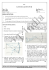### Nivo 2x2 Instructions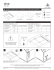### installation of an angle board * * * thermopian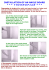### BITSAT Grand Test-3 - sakshieducation.com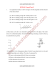### Canon 60D camera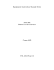### 972-258-6400 | TYLOCK.COM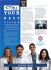### Review12.1-12.6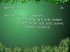### MACRO VISION ACADEMY, BURHANPUR Entrance Test - 2013 -14 Section